Documentation

## Measurement of Pulse and Transition Characteristics

This example shows how to analyze pulses and transitions and compute metrics including rise time, fall time, slew rate, overshoot, undershoot, pulse width, and duty cycle.

### Clock Signal with Noise

First let's view the samples from a noisy clock signal.

```load clocksig clock1 time1 Fs plot(time1, clock1) xlabel('Time (secs)') ylabel('Voltage')```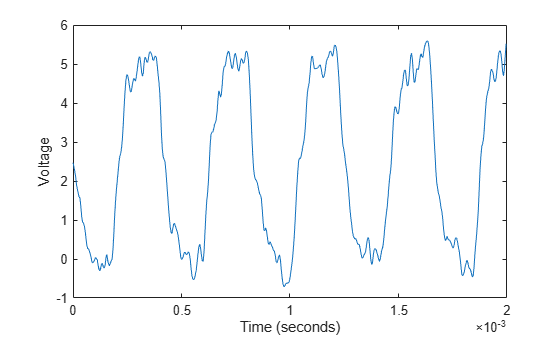### Estimate State Levels

Use `statelevels` with no output argument to visualize the state levels. State levels are estimated via histogram.

`statelevels(clock1)`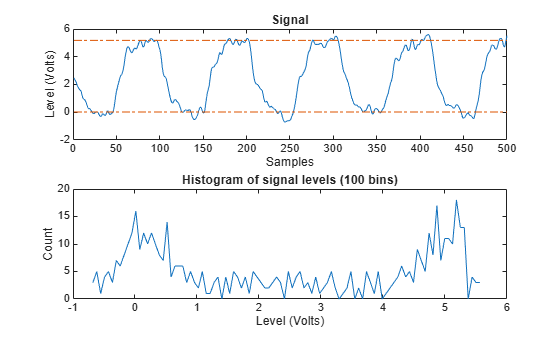```ans = 1×2 0.0138 5.1848 ```

The computed histogram is divided into two equal sized regions between the first and last bin. The mode of each region of the histogram is returned as an estimated state level value in the command window.

Use optional input arguments to specify the number of histogram bins, histogram bounds, and the state level estimation method.

### Measure Rise Time, Fall Time and Slew Rate

Use `risetime` with no output argument to visualize the rise time of positive-going edges.

`risetime(clock1,time1)`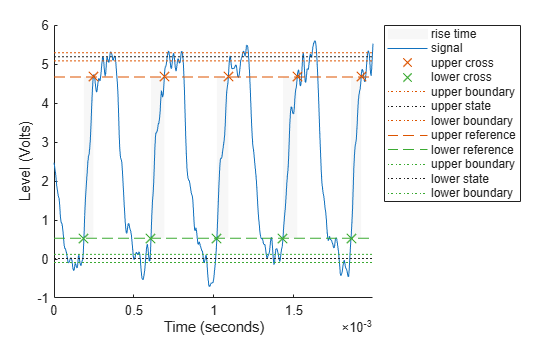```ans = 5×1 10-4 × 0.5919 0.8344 0.7185 0.8970 0.6366 ```

The default reference levels for computing rise time and fall time are set at 10% and 90% of the waveform amplitude.

Specify custom reference and state levels via optional input arguments as shown below for a fall time measurement.

`falltime(clock1,time1,'PercentReferenceLevels',[20 80],'StateLevels',[0 5])`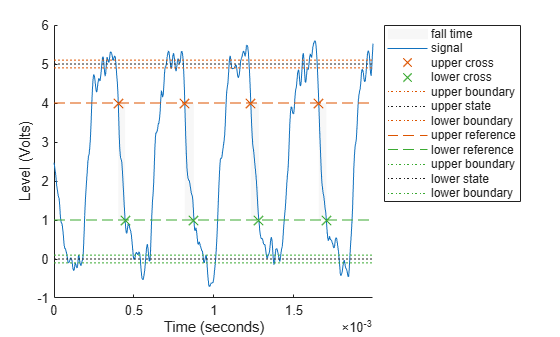```ans = 4×1 10-4 × 0.4294 0.5727 0.5032 0.4762 ```

Obtain measurements programmatically by calling functions with one or more output arguments. For uniformly sampled data, you can provide a sample rate in place of the time vector. Use `slewrate` to measure the slope of each positive-going or negative-going edge.

`sr = slewrate(clock1(1:100),Fs)`
```sr = 7.0840e+04 ```

### Analyze Overshoot and Undershoot

Now let's view data from a clock with significant overshoot and undershoot.

```load clocksig clock2 time2 Fs plot(time2, clock2) xlabel('Time (secs)') ylabel('Voltage')```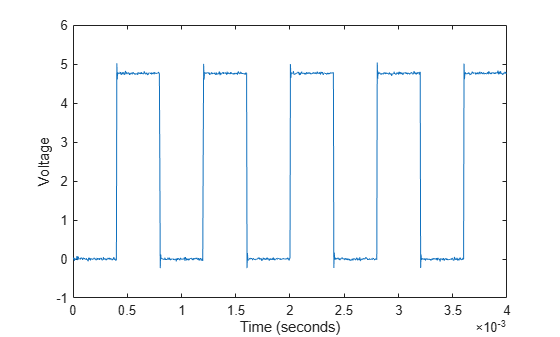Underdamped clock signals have overshoots. Overshoots are expressed as a percentage of the difference between state levels. Overshoots can occur just after an edge, at the start of the post-transition aberration region. These are called postshoot overshoots. You can measure them by using the `overshoot` function.

`overshoot(clock2(95:270),Fs)`
```ans = 2×1 4.9451 2.5399 ```
`legend('Location','NorthEast')`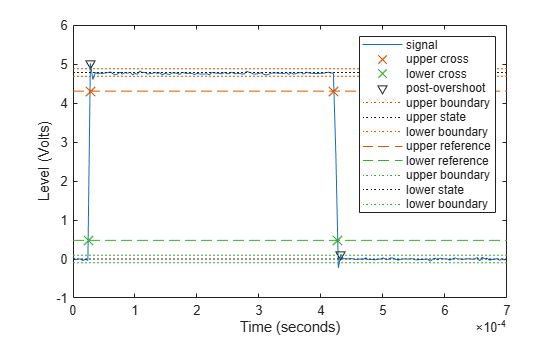Overshoots may also occur just before an edge, at the end of the pre-transition aberration region. These are called preshoot overshoots. Similarly, you can measure undershoots in the pre and post-aberration regions. Undershoots are also expressed as a percentage of the difference between the state levels. Use optional input arguments to specify the regions in which to measure aberrations.

`undershoot(clock2(95:270),Fs,'Region','Postshoot')`
```ans = 2×1 3.8499 4.9451 ```
`legend('Location','NorthEast')`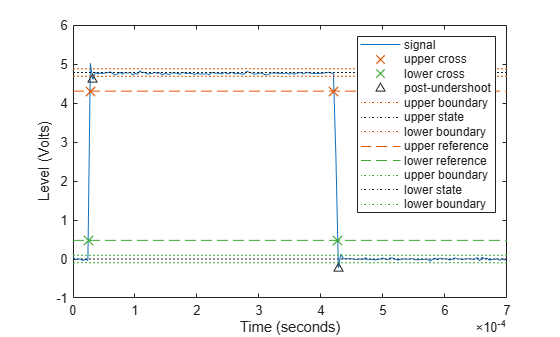### Measure Pulse Width and Duty Cycle

Use `pulsewidth` with no output argument to plot highlighted pulse widths.

`pulsewidth(clock2, time2, 'Polarity', 'Positive');`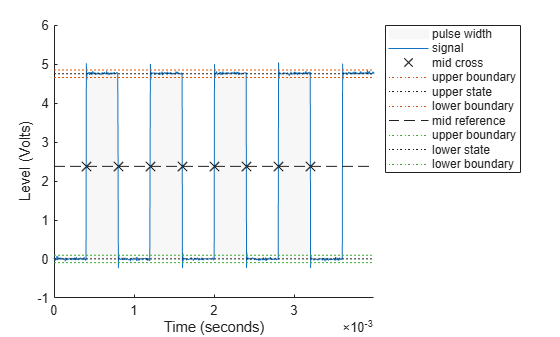This displays pulses of positive polarity. Select negative polarity to see the widths of negative polarity pulses.

Use `dutycycle` to compute the ratio of the pulse width to the pulse period for each positive-polarity or negative-polarity pulse.

`d = dutycycle(clock2,time2,'Polarity','negative')`
```d = 3×1 0.4979 0.5000 0.5000 ```

Use `pulseperiod` to obtain the periods of each cycle of the waveform. Use this information to compute other metrics like the average frequency of the waveform or the total observed jitter.

```pp = pulseperiod(clock2, time2); avgFreq = 1./mean(pp)```
```avgFreq = 1.2500e+03 ```
`totalJitter = std(pp)`
```totalJitter = 1.9866e-06 ```